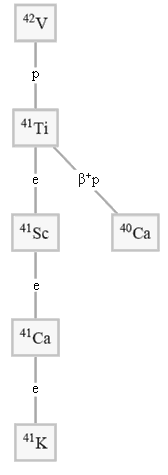The activity,$$A$$, of a radioactive nuclide describes how many decay occurs. The unit Becquerel (Bq) is a measure of decays per second. As nuclide decay, there are fewer left avaible to decay. Thus, the activity decreases with time:

$$A(t) = A_0 \cdot e^{-\lambda \cdot t}$$

$$A(t) = A_0 \cdot e^{-ln(2) \cdot \frac{t}{t_{1/2}}}$$

Given activity is number of decays per second (or the rate of decays) is can be expressed as:

$$A = \lambda \cdot N$$

Another common unit is the Curie (Ci) with the conversion:

$$1 \; [Ci] = 3.7 \cdot 10^{10} \; [Bq]$$

A nonillion ($$10^{30}$$) nuclide of element X decay with a half life of 10 years. What is the activity in Bq and Ci?

## Branching Decays

Radioactive elements may decay through different reactions. A decay scheme shows the path to a stable element. Originally, Vanadium decays into titanium which is also radioactive. Titanium has two decay processes and eventually ends up at stable Potassium.We can add up the decay constants to obtain the total:

$$\lambda_{tot} = \sum_i^n {\lambda_i}$$

A radioactive sample has measured activity of $$2 \; [mCi]$$ and two decay modes $$\lambda_1 = 0.005 \; [s^{-1}]$$ and $$\lambda_2 = 0.0005 \; [min^{-1}]$$. What is the activity in after a minute?